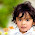### Multilingual Hedge Fund - Combinatorics Puzzle

Source: Quantnet Forums

Problem:
A hedge fund has 70 employees. For any two employees X and Y there is a language that X speaks but Y does not, and there is a language that Y speaks but X does not. At least how many different languages are spoken by the employees of this hedge fund?

Update: (Dec 17, 2012)
Correct answer posted by Nikhil Jain (IT BHU Alumnus 2008), Paul Wong and Tuhin (IITB 4th year student) in comments.

Similar Problem:
A very similar but more difficult problem posted a couple of years back: http://pratikpoddarcse.blogspot.in/2009/12/number-of-locks-and-keys.html

1.2*ceil(log2(70)) ?

2.8 languages.

Maximum number of unique combinations possible with 8 languages is 8c4 which is equal to 70. Thus if every employee knows 4 languages then 8 different languages will be sufficient to meet our case.

3.70..each employee speaks diff language...

4.let x be number of language, max combination when each one takes up x/2 number of language

x C (x/2) >= 70

x = 8

5.The least number of languages that can be spoken are 8. Say the set of of languages be L = {A, B, C, .....}. Then for each of 70 employees, there should be a distinct combination for each of the 70 employees. We see that 8 distinct languages suffices because 8!/4!4! = 70, i.e, each of the 70 employees should choose a distinct 4 set from these 8 distinct languages

6.7
2^7=128 > 70

Either you know the language (think boolean 1) or you do not (think boolean 0). Number of unique combinations needed is more than 70.

(baki aap khud samjhdaar hain :P)

7.Vaibhav, The question asks for "at least" how many?

Correct answer is 8. Thanks Nikhil, Paul and Tuhin for the correct answer.

1.The answer for 71 people should still be 8 but this method gives answer as 9.

Is this approach right?
70 people : Take one language. x people speak it and 70-x don't. Hence the total number of languages required = 1 + Max(total_languages(x),total_languages(70-x)). For least number of languages, x = 70 - x. Hence x = 35

and so on.... after that x= 18.... and then x = 9 ..... then 5... then 3... then 2.... then 1

This approach gives for 71 people total languages = 8

2.This comment has been removed by the author.

3.Please Ignore. I messed up the solution.

8.This is based on Sperner's Theorem.
In this case ans is 8.

9.The question never mentions that each employee must speak the same number of languages. Assuming that they can speak different number of languages, the minimum languages that can be spoken is 6, as Sum[1 to 5]( 6 C i) > 70.